﻿ Calculation method > Line Of Sight

# LINE OF SIGHT

Navegación:  Calculation method > Line Of Sight

# LINE OF SIGHT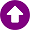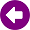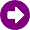DESCRIPTION

The LOS model (Line-Of-Sight model) considers a signal transmitted through free space to a receiver located at a given distance from the transmitter. It assumes there are no obstructions between transmitter and receiver and that the signal propagates along a straight line between the two. If the radio path is obstructed, the resulting received signal level is zero. In other words, no diffraction effects are taken into account by this model.

Actually, the signal trajectory follows a curve, whose bending is caused by the refractive index variations in the troposphere. In order to achieve a representation in terms of rectilinear propagation, the notion of the effective Earth radius is employed, assuming a typical value of 4/3 for the k-factor, though any other value can be used, as defined by the radio link designer. This factor is assumed as a constant parameter for a particular path that modifies the Earth radius, thus modelling the curvature of the signal trajectory

DEVELOPMENT

As it was previously mentioned, a condition of clear visibility is required in order to estimate the basic propagation loss using the LOS model. The clearance analysis is carried out on the basis of the terrain profile of the radio path between transmitter and receiver, verifying that LOS conditions exist between both link ends.

If the path between transmitter and receiver is blocked by the terrain, the received signal level is assumed to be zero. Otherwise, free space propagation is then calculated using any of the following two procedures, recommended by the ITU-R Rec. 525-2 . Both lead to the same results.

The field strength at a point located at a certain distance from the transmitter is calculated by the expression: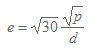(1)

where:

e: root mean square field strengt [V/m]

p: isotropically radiated power (EIRP) of the transmitter in the direction of the point in question [W]

d: distance from the transmitter to the point in question [m]

Equation (1) can be expressed in dBu (dBµV/m):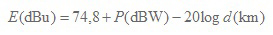(2)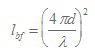(3)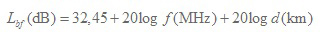(4)